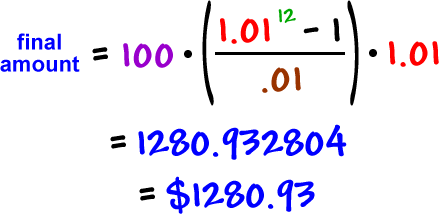Luckily, yes, there IS a formula for finding the value of annuity investments!

Note:  We are making our investments at the beginning of each
period (month in most cases.)  The typical annuity formula that
appears in some Algebra books is for when the investment is
made at the end of the period.  This causes the first month's
interest to be lost and isn't representative of how most people
invest.

Let's invest \$100 each month at 12%*.  How much will we have in one year?

* If we invest each month, we need to assume that the account
will be compounded monthly to use this formula.

Again, we'll use the "growth factor" of money.

investment amount = \$100

growth factor = \$1.01

interest per period = .01

number of periods = 12

Here's the formula:With our numbers:Hey, it works!  We got the same amount that we got doing it the long month-by-month way.